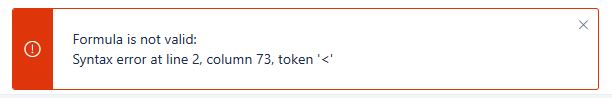# How to use greater than to or equal (>=) and less than or equal to (<=)

CASE
WHEN [Measures].[Issues created] >= 97 and [Measures].[Issues created] =< 100 then ‘Expected’
ELSE 0
ENDIn your formula you mixed up the order, it should be <= not =<

You will then run in another error because you show a string in the THEN-case but a number in the ELSE-case. It should be both string or number.

1 Like

Silly me, how could I not notice that?Thanks @sven!